46
Solving Linear Recurrence Relation

### Definition :

A linear recurrence relation is a function or a sequence such that each term is a linear combination of previous terms. Each term can be described as a function of the previous terms.

A famous example is the Fibonacci sequence: f(i) = f(i-1) + f(i-2)

Linear means that the previous terms in the definition are only multiplied by a constant (possibly zero) and nothing else. So, this sequence f(i) = f(i-1) * f(i-2) is not a linear recurrence.

### Problem

Given a function f(x) defined as a linear recurrence relation. Compute f(N) ,where N is a large number .

### Solution

The solution is broken down into 4 steps :

1. Determine K , the number of terms on which f(i) depends

K is the minimum integer such that f(i) doesn’t depend on f(i-M), for all M > K.
For Fibonacci sequence, because the relation is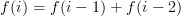therefore, K = 2.

2. Determine vector F1, the initial values

If each term of a recurrence relation depends on K previous terms, then it must have the first K terms defined, otherwise the whole sequence is undefined. For Fibonacci sequence (K = 2), the well-known initial values are:

f(1)=1 , f(2)=1

We define a column vector Fi as a K x 1 matrix whose first row is f(i), second row is f(i+1), and so on, until Kth row is f(i+K-1). The initial values of f are given in column vector F1 that has values f(1) through f(K):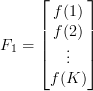3. Determine T, the transformation matrix

This is the most important step in solving recurrence relation. The heart of this method is to construct a K x K matrix T, called transformation matrix, such that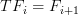Here is how to construct it. Suppose that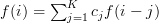.

You can solve this equation with any method, and obtain the result: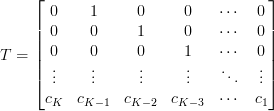More precisely, T is a K x K matrix whose last row is a vector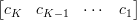, and for the other rows, the ith row is a zero vector except that its (i+1)th element is 1.

Let’s apply it to our example. In Fibonacci sequence,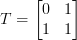4. Determine FN

After we construct the transformation matrix, the rest is very simple. We can obtain Fi for any i, by repeatedly multiplying T with F1. For example, to obtain F2,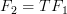To obtain F3,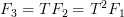And so on. In general,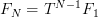Therefore, the original problem is now (almost) solved: compute FN as above, and then we can obtain f(N): it is exactly the first row of FN. In case of our Fibonacci sequence, the Nth term in Fibonacci sequence is the first row of: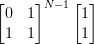What remains is how to compute TN-1 efficiently. The most popular method is to use exponentiation by squaring method that works in O(log N) time, with this recurrence:

•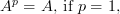•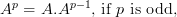•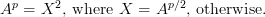Multiplying two matrices takes O(K3) time using standard method, so the overall time complexity to solve a linear recurrence is O(K3 log N).

Here is a sample C++ code to compute the N-th term of Fibonacci sequence, modulo 1,000,000,007.

#include<iostream>
#include<vector>
#define REP(i,n) for (int i = 1; i &lt;= n; i++)
using namespace std;

typedef long long ll;
typedef vector<vector<ll> > matrix;
const ll MOD = 1000000007;
const int K = 2;

// computes A * B
matrix mul(matrix A, matrix B)
{
matrix C(K+1, vector<ll>(K+1));
REP(i, K) REP(j, K) REP(k, K)
C[i][j] = (C[i][j] + A[i][k] * B[k][j]) % MOD;
return C;
}

// computes A ^ p
matrix pow(matrix A, int p)
{
if (p == 1)
return A;
if (p % 2)
return mul(A, pow(A, p-1));
matrix X = pow(A, p/2);
return mul(X, X);
}

// returns the N-th term of Fibonacci sequence
int fib(int N)
{
// create vector F1
vector<ll> F1(K+1);
F1 = 1;
F1 = 1;

// create matrix T
matrix T(K+1, vector<ll>(K+1));
T = 0, T = 1;
T = 1, T = 1;

// raise T to the (N-1)th power
if (N == 1)
return 1;
T = pow(T, N-1);

// the answer is the first row of T . F1
ll res = 0;
REP(i, K)
res = (res + T[i] * F1[i]) % MOD;
return res;
}